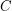# Cycle double cover conjecture

 Importance: Outstanding ✭✭✭✭
 Author(s): Seymour, Paul D. Szekeres, George
 Subject: Graph Theory » Basic Graph Theory » » Cycles
 Keywords: cover cycle
 Recomm. for undergrads: no
 Prize: none
 Posted by: mdevos on: March 7th, 2007
Conjecture   For every graph with no bridge, there is a list of cycles so that every edge is contained in exactly two.

This beautiful conjecture was made independently by Szekeres and Seymour in the 70's and is now widely considered to be among the most important open problems in graph theory. Note the similarity between this conjecture and the Berge-Fulkerson conjecture on perfect matchings. Attempts to prove this conjecture have lead to a variety of conjectured strengthenings, which appear on other pages. See: The circular embedding conjecture, The five cycle double cover conjecture, The faithful cover conjecture, and Decomposing Eulerian graphs.

If a graphhas a nowhere-zero 4-flow then it follows from a result of Tutte thatsatisfies the above conjecture. Thus, by Jaeger's 4-flow theorem [J], the above conjecture is true for every 4-edge-connected graph. A cubic graph has a nowhere-zero 4-flow if and only if it is 3-edge-colorable, so the above conjecture is also true for 3-edge-colorable cubic graphs. In general, it follows from vertex splitting arguments that problem may be reduced to cubic graphs which are not 3-edge-colorable.

For a general graphwith no cut-edge, Bermond, Jackson and Jaeger [BJJ] used Jaeger's 8-flow theorem [J] to prove thathas a list of circuits so that every edge is contained in exactly four. Fan [F] used Seymour's 6-flow theorem [S81] to prove that G has a list of circuits so that every edge is contained in exactly six.

Letbe a directed graph and letbe a circuit (not necessarily a directed circuit) of. If we choose a direction to travel around, then every edge ofis either traversed forward or backward. The following strengthening of the cycle double cover conjecture takes directions into account.

Conjecture  (The oriented cycle double cover conjecture)   Ifis an orientation of a bridgeless graph, then there is a listof circuits ofwith directions so that every edge ofis traversed forward by exactly one circuit inand backward by exactly one circuit in.

Tutte also showed that every graph with a nowhere-zero 4-flow satisfies this conjecture. Thus, as above this conjecture is true for 4-edge-connected graphs and for 3-edge-colorable cubic graphs.

It was mentioned above that for a general graphwith no bridge, there is a list of circuits containing every edge exactly four times. By taking two copies of each circuit in this list and giving them opposite directions, we have a list of circuits so that every edge is traversed forward and backward exactly four times. Luis Goddyn and I (M. DeVos) have observed that the same ideas used in Fan's article [Fa] can be used to construct a list of circuits with directions so that every edge is traversed forward and backward exactly three times. The following natural question seems to be open.

Conjecture  (The oriented cycle four cover conjecture)   Ifis an orientation of a bridgeless graph, then there is a listof circuits ofwith directions so that every edge ofis traversed forward by exactly two circuits inand backward by exactly two circuits in.

Since every graph with a nowhere-zero 4-flow has a list of circuits with directions traversing every edge forward and backward exactly once, the above conjecture would follow from The three 4-flows conjecture.

## Bibliography

[AGZ] B. Alspach, L. Goddyn, and C-Q Zhang, Graphs with the circuit cover property, Trans. Amer. Math. Soc., 344 (1994), 131-154. MathSciNet

[BJJ] J.C. Bermond, B. Jackson, and F. Jaeger, Shortest covering of graphs with cycles, J. Combinatorial Theory Ser. B 35 (1983), 297-308. MRhref{0735197}

[DJS] M. DeVos, T. Johnson, P.D. Seymour, Cut-coloring and circuit covering

[F] G. Fan, Integer flows and cycle covers, J. Combinatorial Theory Ser. B 54 (1992), 113-122. MathSciNet

[FZ] G. Fan and C.Q. Zhang, Circuit decompositions of Eulerian graphs, J. Combinatorial Theory Ser. B 78 (2000), 1-23. MathSciNet

[FG] X. Fu and L. Goddyn, Matroids with the circuit cover property, Europ. J. Combinatorics 20 (1999), 61-73. MathSciNet

[J] F. Jaeger, Flows and Generalized Coloring Theorems in Graphs, J. Combinatorial Theory Ser. B 26 (1979) 205-216. MathSciNet

[Ki] P.A. Kilpatrick, Tutte's First Colour-Cycle Conjecture, Thesis, Cape Town (1975).

[Sz] G. Szekeres, Polyhedral decompositions of cubic graphs. Bull. Austral. Math. Soc. 8, 367-387. MathSciNet

[S91] P.D. Seymour, Nowhere-Zero 6-Flows, J. Combinatorial Theory Ser. B 30 (1981) 130-135. MathSciNet

[S79] P.D. Seymour, Sums of circuits in Graph Theory and Related Topics edited by J.A. Bondy and U.S.R. Murty, Academic Press, New York/Berlin (1979), 341-355. MathSciNet

[S95] P.D. Seymour, Nowhere-Zero Flows, in Handbook of Combinatoircs, edited by R. Graham, M. Grotschel and L. Lovasz, (1995) 289-299. MathSciNet

[T54] W.T. Tutte, A Contribution on the Theory of Chromatic Polynomials, Canad. J. Math. 6 (1954) 80-91. MathSciNet

[T66] W.T. Tutte, On the Algebraic Theory of Graph Colorings, J. Combinatorial Theory 1 (1966) 15-50. MathSciNet

* indicates original appearance(s) of problem.

### Claimed solution in the affirmative

Alexander Souza has offered for review on arXiv a constructive solution in the affirmative.

http://arxiv.org/abs/1202.0569

Edit: Unless we are mistaken, the Petersen graph is a counterexample to Theorem 2. Furthermore there is an unresolved problem with Lemma 9.

### question

should the cycles be distinct??

### No; consider the example of

No; consider the example of a graph which is itself a cycle. This has a unique cycle double cover, and the cycles are not distinct.

## Comment viewing options

Select your preferred way to display the comments and click "Save settings" to activate your changes.